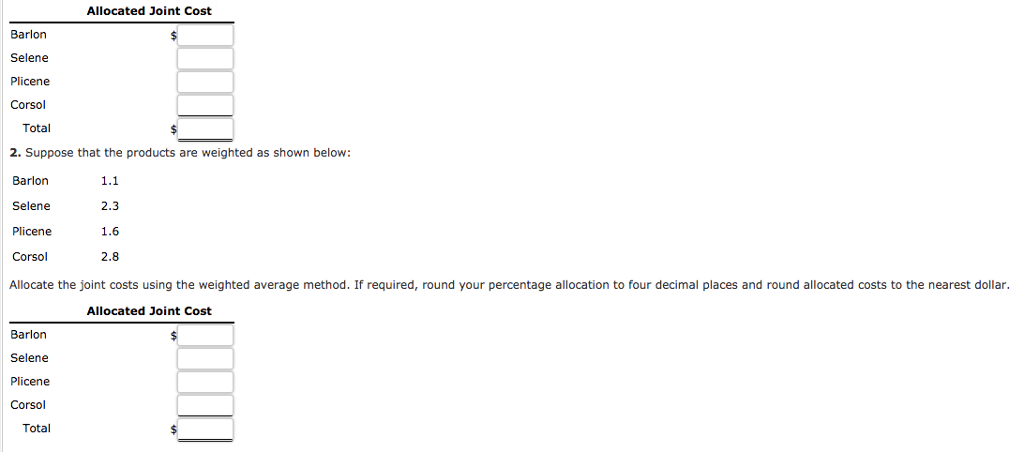# Question & Answer: Allocated Joint Cost Barlon Selene Plicene Corsol Total 2. Suppose that the products are weighted a…..

Alomar Company manufactures four products from a joint production process: barlon, selene, plicene, and corsol. The joint costs for one batch are as follows:

 Direct materials \$69,831 Direct labor 35,261 Overhead 25,501

At the split-off point, a batch yields 1,560 barlon, 3,901 selene, 3,511 plicene, and 4,032 corsol. All products are sold at the split-off point: barlon sells for \$14 per unit, selene sells for \$21 per unit, plicene sells for \$25 per unit, and corsol sells for \$36 per unit.

Don't use plagiarized sources. Get Your Custom Essay on
Question & Answer: Allocated Joint Cost Barlon Selene Plicene Corsol Total 2. Suppose that the products are weighted a…..
GET AN ESSAY WRITTEN FOR YOU FROM AS LOW AS \$13/PAGE

Required:

1. Allocate the joint costs using the physical units method. If required, round your percentage allocation to four decimal places and round allocated costs to the nearest dollar. Note: The total of the allocated cost does not equal to the one provided in the question data due to rounding error.Allocated Joint Cost Barlon Selene Plicene Corsol Total 2. Suppose that the products are weighted as shown below: Barlon Selene Plicene Corsol Allocate the joint costs using the weighted average method. If required, round your percentage allocation to four decimal places and round allocated costs to the nearest dollar 1.1 2.3 1.6 2.8 Allocated Joint Cost Barlon Selene Plicene Corsol Total

The total of joint cost = Material + labor + Overhead = 130,593

Phisical weights method : Total Joint cost shall be shared in the ratio of weights or units obtained for each product. i.e more quantity of output, more will be share of joint cost and like that.

Barlon = 130,593 * 1560 / 13004 = 15666

Note ….1560/13004 …………represents ratio of ……..[Barlon units / Total units of all Four products]

Selene = 130,593 * 3901/13004 = 39,176

Picene = 130,593 * 3511/13004 = 35,259

Corsol = 130,593 * 4032/13004 = 40,492

Total = …………………………………… 130,593

Weighted average method : This is also similar to physical weights method. But ratio of quantity shall be taken after multiplying with respective weights. That means relative importance of the product is attached to physical weights.

 Product Units Weight U * W Ratio Joint cost Barlon 1560 1.1 1716 0.0622 8123 Selene 3901 2.3 8972.3 0.3251 42456 Plicene 3511 1.6 5617.6 0.2036 26589 Corsol 4032 2.8 11289.6 0.4091 53425 27595.5 130593

In the above table …….last column represents the final answer of joint cost allocation for each product.

Ratio = Individual U*W / Total of U*W………..For example for Barlon = 1716 / 27595.6 = 0.0622

Joint cost of Barlon = 130593 * 0.0622, …… for Selene = 130593 * 0.3251………..so on for remaining products.